Name:    2nd Grade Operations and Algebraic Thinking- Work with equal groups of objects to gain foundations for multiplication, Standard 4, Pre Test 1 Use addition to find the total number of objects arranged in rectangular arrays with up to 5 rows and up to 5 columns; write an equation to express the total as a sum of equal addends.

Multiple Choice
Identify the choice that best completes the statement or answers the question.

1.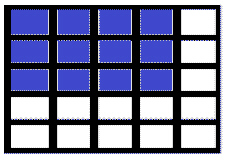Which equation below describes the colored portion of the array?
 a. 5 + 5 + 5 c. 3 + 3 + 3 b. 4 + 1 + 4 + 1 d. 4 + 4 + 4

2.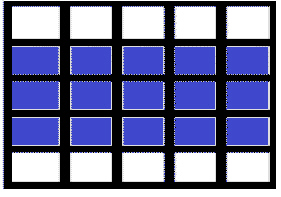Which equation below describes the colored portion of the array?
 a. 5 + 5 + 3 c. 3 + 3 + 3 + 3 + 3 b. 5 + 5 + 5 d. 4 + 4 + 4

3.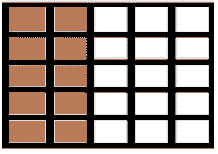Which equation below describes the colored portion of the array?
 a. 2 + 2 + 2 + 2 + 2 c. 3 + 3 + 3 + 3 + 3 b. 5 + 5 + 5 + 5 + 5 d. 1 + 1

4.Which equation below describes the colored portion of the array?
 a. 2 + 2 + 2 + 2 + 2 c. 3 + 3 + 3 + 3 + 3 b. 5 + 5 + 5 + 5 + 5 d. 1 + 1

5.Which equation below describes the colored portion of the array?
 a. 2 + 2 + 2 + 2 + 2 c. 3 + 3 + 3 + 3 + 3 b. 4 + 4 + 4 + 4 d. 1 + 1 + 1 + 1

6.

Which array below describes this equation:  3 + 3 + 3?
 a.c.b.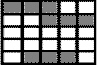d.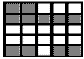7.

Which array below describes this equation:  2 + 2 + 2?
 a.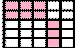c.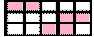b.d.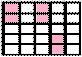Completion
Complete each statement.

8.

Write an equation that shows the sum of the colored squares in the array below.  Put a space between each number and symbol.  Don’t forget the = sign and the answer.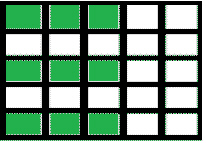9.

Write an equation that shows the sum of the colored squares in the array below.  Put a space between each number and symbol.  Don’t forget the = sign and the answer.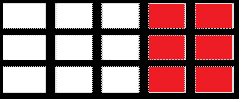10.

Write an equation that shows the sum of the colored squares in the array below.  Put a space between each number and symbol.  Don’t forget the = sign and the answer.